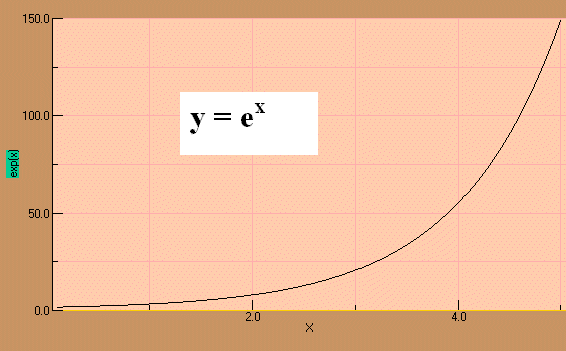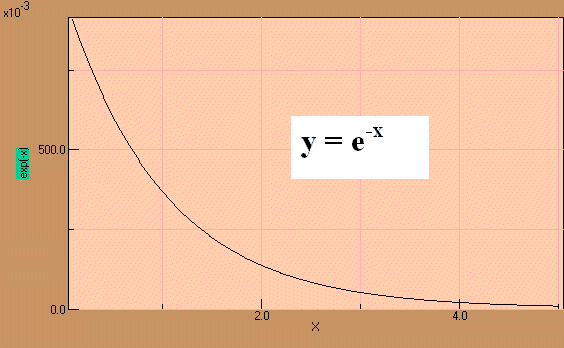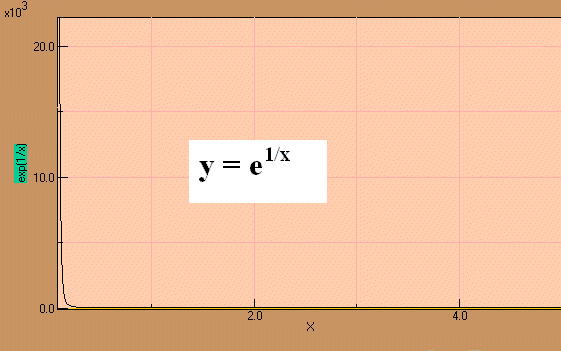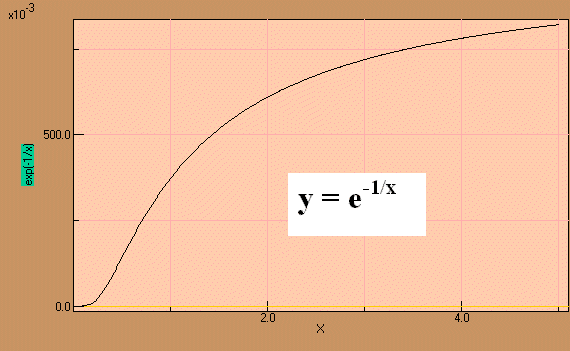# Simple Exponential functionsWith this JAVA module you can plot exponential (or other) functionsHer are a few examples for writing equations
cos(x) exp(x)
cos(x*2)   exp(1/x)
cos(x/40)   x^2
cosh(x)   1/x^2
cosh(40*x)   1/(x^3+10)
1/(exp(x-20)+1)Here are a few results for exponentials:2.4.1 Special Applications